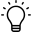# Determination of PID parameters using table methodW dokumencie opisane są tabelaryczne metody doboru nastaw w oparciu o model inercyjny pierwszego rzędu. Parametry modelu wyznaczane są z użyciem narzędzia Realtime Monitor FunctionSummary:
This document describes PID tuning using step response method. Settings are calculated base on a first order inertia model, where a model constatns are approximated using the GX Log Viewer graph

# 1. Realtime Monitor settings

To determine the waveform parameters, it will be necessary to add the control value (CV) and the process value (PV) to the monitoring. An set value (SV) can also be included to check the operation of the controller.Realtime Monitor functions introduction:  Real Time Monitor Function guide

# 2. Determination of model

### 2.1. Step response

Set the value of the control signal to the level corresponding to the operating point and wait for the value to settle. Then increase the value of the control signal, e.g. by 10-20%. In the case of simple systems (e.g. heating plate temperature control), it is possible to make a jump from zero to the operating point.### 2.2. Determination of gain

Position the blue cursor just before the CV spike occurs. The red cursor should be set where the measured value reaches a constant value. Click on the waveform of the measured value and read the Difference value (Blue->Red), which corresponds to the increment of the measured value (dPV).dPV=999
By analogy, you can measure the gain of the control signal (dCV), but it is already known:dCV=200
The gain K of the object is approximately:K=dPV/dCV=999/200 =5
Time constant values will be calculated depending on the method.

### 2.3. T95 method

The method is based on the measurement of the delay time and the time after which the measured value reaches 95% of the value in the steady state (relative to the initial value). The next steps are shown in the diagrams.

Delay measurement - set the first cursor at the moment of the control value jump, and the second one at the moment when the measured value starts to increase. The measured time can be read in the Span field (Blue->Red).Top=0.86

The time constant is estimated as 1/3 of the time value from the beginning of the measured value increase (after the delay time has elapsed) to the moment when the measured value reaches 95 values in the steady state after the control jump:T=1/3*10.45=3.48

### 2.4. Secant method

In the secant method, the delay time and the time constant of the object are determined based on two time measurements:t1=4.09t2=5.11

The values of the time constant and delay time can be calculated from the following formulas:Top=(t1-t2ln2)/(1-ln2)=(t1-t2*0.693)/0.307=0,31T=t2-Top=4,80

# 3. Regulation constants calculation

Based on the knowledge of the object gain K, the time constant T and the delay time Top, the PID controller settings can be calculated based on the setting tables. This is one of many examples available in the literature.

 Overshoot Regulator kp Ti Td 0% P 0.3*T/(K*Top) - - PI 0.6*T/(K*Top) 0.8*Top+0.5*T - PID 0.95*T/(K*Top) 2.4*Top 0.4*Top 20% P 0.7*T/(K*Top) - - PI 0.7*T/(K*Top) Top+0.3*T - PID 1.2*T/(K*Top) 2*Top 0.4*Top

For example, for the model parameters calculated with the T95 method and 0% overshoot, we will obtain the PID controller settings:kp=0,77
Ti=2,06
Td=0.344

In the PID parameters, the gain values are given in percent, the integral action time constant in 100 millisecond increments, and the derivative action time constant in 10 millisecond increments:In order to enter the calculated settings correctly, the following dependencies must be taken into account:KP=100*kp=77
TI=10*Ti=21
TD=100*Td=34

An example of a response to a jump disturbance:The obtained constants may be far from optimal. The method can be useful for rough determination of regulation constants, e.g. when there are problems with the autotuning function.